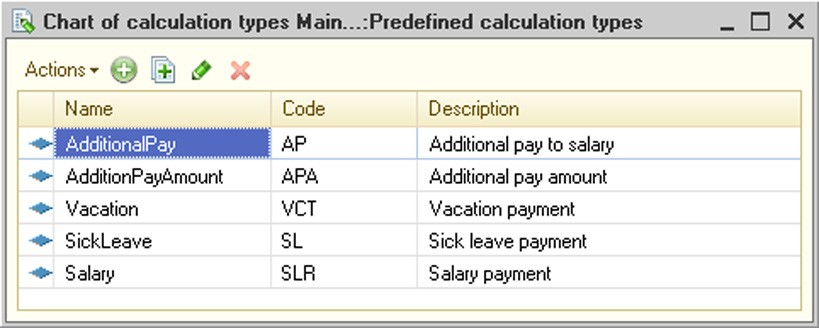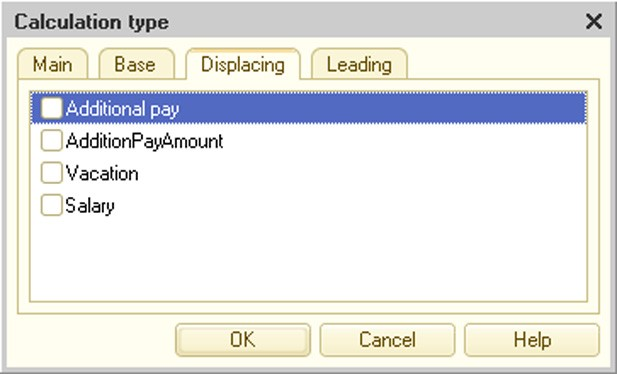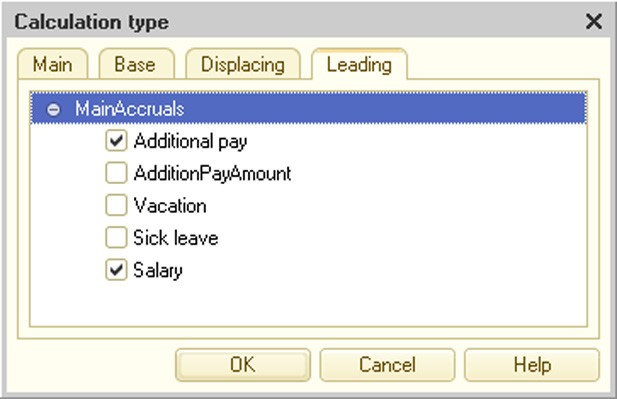# PERIODICAL CALCULATIONS

Periodical calculations are calculations made with a certain frequency; they are closely bound one to another based on a number of rules and affect each other within a period.

The mechanisms of periodical calculations allow you to set up their order and correlation and organize the recording of their results.

Calculation of salary that computes accruals and withholdings is a typical example of a periodical calculation. Calculations are usually performed on a monthly basis and the results of one calculation can depend on the results and availability of other calculations.

The Charts Of calculation types and Calculation registers configuration objects are used for calculations in 1C:Enterprise. Since these objects are closely connected, this chapter outlines the features they provide.

The subsequent sections discuss the issues of configuring the mentioned objects.

## 12.1. MAJOR CONCEPTS

Period. The concept of period is important for calculations. Typically, a period is described by the date it begins and the date it ends. If periodicity is defined for a calculation, describe the period of calculation validity or registration by indicating any date. This date is used to identify the period's start date which also describes this period. Defining a period in this fashion optimizes execution of queries that select records within the specified period.

Calculation periodicity. It defines the period over which calculations recorded in a particular register are (can) be performed. It is specified in the calculation register's Periodicity property. The value of this property determines the period of register record validity (if the register is periodical). For example, if the register periodicity is Month, the document date (e.g., November 2008) is selected as the action period for record generation and this date is used by the system to assign 11/01/2008 as the action period's start date.

Registration period is the start date of the period indicated when registering the calculation (it is defined on the basis of the recorder document date). Assume salary for May 2008 is calculated in June 2008 (calculation periodicity: Month). May 2008 is the action period (the date 05/01/2008 is entered in the database), while June 2008 is the registration period (the date 06/01/2008 is entered in the database).

Action period is the period's start date determined based on the value of the Periodicity property. Assume the document points out that calculation is performed for May 2008. The action period for the Periodicity property of the Month register is defined by the date 05/01/2008; for the Quarter register – by 04/01/2008.

Calculation action period indicates the period over which the calculation is made. The period is defined by its beginning and end dates. Assume a sick-list record for May 2008 has an action period of 05/01/2008; in this case the calculation action period is defined by the beginning date (e.g., 05/06/2008) and the end date (e.g., 05/15/2008).

Displacement mechanism or action period competition is discovering the connections between calculation types during the calculation action period. Competition results from inability to run multiple calculations at a time. You need to select the calculation to be performed in this period. The displacement mechanism is set up in the description of a specific calculation type. You can adjust these settings in the Displacing section (for calculation types). For example, Salary Payments and Sick Leave Payments cannot be applied at the same time. This means that the sick leave payment displaces the salary payments. In other words, when the sick leave is active, the salary is not calculated. For a description of the displacement mechanism see page 1-668.

Actual action period – if a calculation is not displaced by other calculations, the actual action period is equal to the action period. If there are displacing calculation types, the actual action period is defined as a combination of non-overlapping periods during which this calculation is not displaced. fig. 270 below shows a graphical representation of an actual action period when the Salary calculation is displaced by the Sick Leave calculation.

The actual action period represents secondary data, i.e. the result of system calculations. The actual action period is recalculated with the entry of any set, including an empty one (posting undone).

IMPORTANT!

Calculation results do not depend on the data entry (registration) sequence.

Basic period defines the period where (basic) calculation results used for the current calculation are selected. For example, when the premium pay for May 2008 is accrued, the results of the accruals performed by certain calculation types

over a certain period (e.g., Salary, Additional Pay, Vacation Pay) are taken into account. This is the basic period for the premium pay calculation. The link between calculation types by basic period is set up in the description of a specific calculation type in the Base section (calculation types).Fig. 270. Displacement Mechanism

## 12.2. CHARTS OF CALCULATION TYPES

These configuration objects are designed to create calculation types used in calculation registers.

A reference to a calculation type is one of the main properties of the calculation register records, rendering different and diverse calculation register entries.

Use the editing window (see page 1-59) or the properties palette to edit the Chart of calculation types object properties and create subordinate objects. The unique properties of charts of calculation types are discussed below in addition to their general properties.

## 12.2.1. Calculation Property Category

Dependence on base calculation type – if the Dependence on base calculation typr property value is not Does not depend, then the list of basic calculation types can be specified for a chart of calculation types. The list of basic calculation types is defined by the Base charts of calculation types property.

Base charts of calculation types – specifies a list of calculation types that can be included in the list of basic calculation types. The list of basic calculation types is used by the calculation register in the base retrieval mechanism.

Uses action period if this property is set, the current chart of calculation types can be assigned to a calculation register with an action period. Moreover, a list of displacing calculation types can be specified for each calculation type. The list of displacing calculation types determines how the displacement mechanism works for a particular calculation register (see page 1-668).

The properties specified in this category define what attributes are included in the list when calculation type forms are created. If the Dependence on base calculation type property is set to Depends by action period (Depends by registration period), you can place a table box for basic calculation type selection in the form. If the Uses action period property is set, a table box for displacing calculation type selection can be placed in the form.

## 12.2.2. Predefined Calculation Types

Plans of calculation types have a special feature: predefined calculation types created at the configuration stage. The user cannot delete them in the 1C:Enterprise mode.

To edit the list of predefined calculation types, go to the properties palette, open the Other tab and click Predefined. The list of predefined calculation types for this chart of calculation types appears (for an example, see fig. 271).Fig. 271. Predefined Charts of Calculation Types

The list of predefined calculation types can be edited using the Actions menu items.

Attributes (basic and leading) of predefined calculation types depend on the properties set for this chart of calculation types object in the Calculation category.

Each predefined calculation type is edited in the modal window. The main properties of a calculation type are set in the Main tab.Fig. 272. Editing Chart of Calculation Types

If the Dependence on base calculation type property is set to Depends by action period (Depends by registration period), the form contains the Base tab. This tab lists the calculation types that are basic for the current calculation type and define how the base retrieval mechanism works for a particular calculation register (see fig. 273).

Thus, in the example below (see fig. 273), Salary and Additional pay calculation types are basic for the Sick leave calculation type.Fig. 273. Basic Accruals for Calculation Type

If the Uses action period property is set, the form contains the Displacing tab. It lists the calculation types displacing the current calculation type over the action period and thus defining how the displacement mechanism works for a particular calculation register.Fig. 274. Displacing Calculation Types

A displacing calculation type should be selected from the listed calculation types (see fig. 274). In the example above, no displacing calculation types exist the Sick leave calculation type. However, when describing the Salary calculation, select Sick leave.

The Leading tab lists the calculation types defining how the recalculation mechanism work for a particular calculation register (i.e., the register this list of calculation types is assigned to).Fig. 275. Leading Calculation Types

In the 1C:Enterprise mode, the user can create custom calculation types in addition to the predefined types and set the necessary properties for them. To do so, a form is created in the Designer in which the table boxes for basic, leading and displaced calculation types, depending on the properties set for this list of calculation types, should be placed.

12.3.          CALCULATION REGISTERS

## 12.3.1. General Information on Calculation Registers

A calculation register is a configuration object that ensures recording of computation results performed by calculations from the chart of calculation types. For example, calculation registers enable accruals for individuals (salary, sick pay, vacation pay and so on).

Calculation register entries can affect the status of other register entries. There are two types of mutual impact exhibited by accounting records in the calculation register: competition for the action period and interdependence in the base period. Let us illustrate these mutual impacts.

## 12.3.1.1. Displacement Mechanism

Action period competition can be illustrated by salary and vacation pay accruals for an individual. The same period of time cannot cover both a salary entry and a vacation pay entry, i.e. the periods of time for which these accruals are made cannot intersect. This kind of entry (register record) behavior is implemented in the calculation register using the concept of register record action period. Every calculation register record has an actual action period usually determined by a set of multiple periods of time.

Thus, if a vacation pay entry is valid from 03/03/2008 to 03/13/2008, a salary accrual entry can, for example, have the actual action period consisting of two intervals: from 03/01/2008 to 03/02/2008 and from 03/14/2008 to 03/20/2008.

Action period competition is supported by the calculation register displacement mechanism, whose operation is defined by the displacing calculations, action period dates, etc.

## 12.3.1.2. Recalculation Mechanism

The base action period dependency for entries can be illustrated by salary accruals and salary-dependent average earnings for an individual. If the status of salary entries in the register changes for a period (entries are deleted, modified or added), the results for average earnings entries are also adjusted if they exist in the period. To implement this kind of mutual impact of calculation register entries, the concept of calculation register base period is introduced. Thus, if the average earnings entry has a base period between 01/01/2008 and 03/31/2008 (i.e. it uses the three-month average earnings), then any change in the salary entries for the same period cause the average earnings entry to be modified.

### 12.3.2. Editing Calculation Register

The Calculation registers configuration tree branch is designed to manage calculation registers.

Editing a calculation register includes specifying a list of calculation types, action period and base period support and periodicity; developing the register structure (sets of dimensions, resources and attributes); creating screen forms and print forms for viewing register records (if necessary).

NOTE

Such values as UUID, BinaryData, and any unlimited length string cannot serve as the type of accounting register dimensions.

The unique calculation register properties are discussed in this section in addition to general object properties.

Use the editing window to edit properties of Calculation register objects and create subordinate objects (see page 1-59).

Chart of calculation types is the main characteristic of a register. For details see page 1-665. Only one chart of calculation types can be specified for a calculation register.

Action period – setting this property creates a mutual competitive impact of register records. Salary accrual and sick pay are a good example of competitive records – you cannot be sick and work at the same time, i.e. get both the salary and sick pay. These calculations are mutually exclusive for each point in time and the system must guarantee that entering one of them excludes the other.

An action period based on the calculation register limits the number of records with similar dimension values, registration periods, and calculation types.

When an actual action period is evaluated (which is performed for each entry of the register record set), a memory shortage error may occur if a large number of similar entries are used in calculation. What this means in real terms is that the number of records active during one and the same time period is limited.

For instance, one and the same employee could have been issued a salary for a single period multiple times.

The limitation value depends on a number of conditions (the database engine used, computer technical parameters, etc.), but under no circumstances does this limitation impact real practice.

Schedule – this property is available if the Action period property is set. This property provides a link to the information register where a time schema for the source data of this calculation is described. A schedule should be specified for the calculations, depending on the source data distributed within the action period, according to a certain rule. For example, it can be an organizational timesheet broken down into days, a lecture schedule in hours, etc.

Schedule value – this property is available if the Action period property is set. This property is used to select a resource of the information register defined in the Schedule property.

For example, the OrganizationWorkSchedule information register can be specified as a schedule. This register has the attributes WorkDay (Boolean type) and WorkHours (Number type). The former shows whether the current date is a workday; the latter specifies the number of work hours. Selecting WorkDay means that the calculation analyzes whether a certain day of the period is a work day (i.e., whether or not salary should be calculated for this day). Selecting WorkHours means that the specified calculation is performed on the basis of the number of working hours. The allocation itself is set in the 1C:Enterprise mode (manually or using the 1C:Enterprise script).

Schedule date – this property is available if the Action period property is set. This property specifies a Date-type dimension of the information register defined in the Schedule property. Depending on the property value, binding to values of the information register resource selected in the Schedule value property is created.

Base period – setting this property creates a mutual coherent impact of the register records. The dependency of pay calculated by averages on the pay calculated in the base period is a good example of coherent register records.

Periodicity defines the period in which records are registered and can affect each other (for registers supporting an action period).

Recalculations are subordinate register objects that define the rules of register records' mutual impact. The register's Dimension property in the Link group of the object property palette indicates the main dimension of the current register that is to be recalculated in case of changes of the leading register data defined in the leading registers' Data property. For example, an individual's withholdings are recalculated if accruals (salary, premium pay) change.

If the Base period property is set, recalculation data are created automatically. If the property is not set the user must create the recalculation data manually (a special form to enter recalculations and their mechanism should be developed at the design stage).